# Team:Tongji Shanghai/Model

Tongji_Shanghai-2016.igem.org Tongji Shanghai

## Achievment

Why we deserve a medal.

### Overview

#### Drug Concentration Forecasting in Body Tissue

In pharmaceutical research and development,it is certainly worth making predictions of trends and variation of the drug concentration in the body tissue. We use a classical pharmacokinetic model to build a drug concentration forecasting function to describe the drug time-dependent concentration change trend, which could be a reference for pharmaceutical research.

### Model Building

I.After taking drug, the change rate of drug concentration in body can be expressed as the dynamics equations: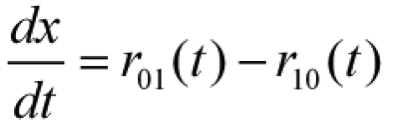where x(t) is the drug concentration in body fluids,
r01(t) is the drug absorption rate,
r10(t) is the elimination rate.

As r10(t) is proportional to the x(t), there is another formula: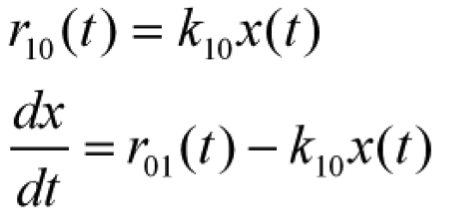where k10 is the constant of elimination rate

II. According to knowledge of pharmacology, absorption rate of drug in proportion to the amount of drug that are not absorbed;the elimination rate in proportion to the drug concentration in body fluids, there is another formula: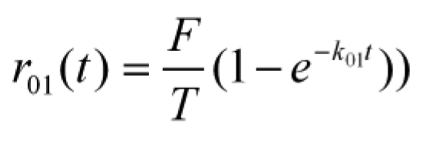F is defined as the total amount of drug.
T is the total time that patients take the drug.
In most cases, drug is taken at moment, T = 0
After several mathematical derivation, we get the model function to describe concentration in body tissue.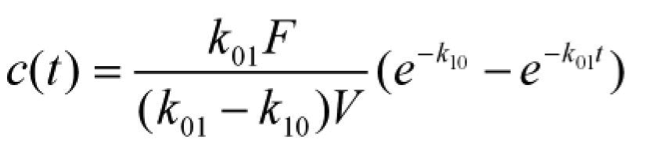### Experiments Verification

We used a set of experiment data to conduct verification of our model.
We measured the drug concentration, after patients take 3g drug sample. And we get the drug concentration change trend in a few hours.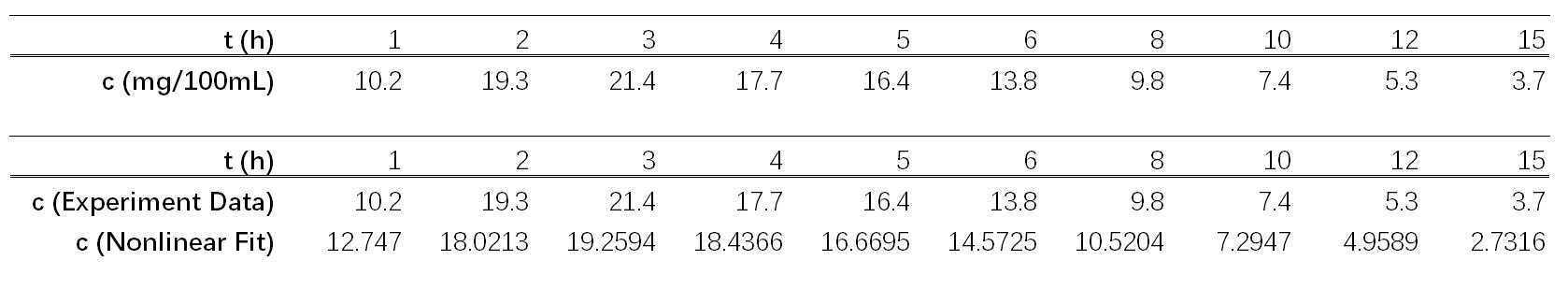Based the experiment data, we use a classical non-linear model to build a differential equation and solve it, estimating the return model parameter from the experimental data by ordinary least squares method.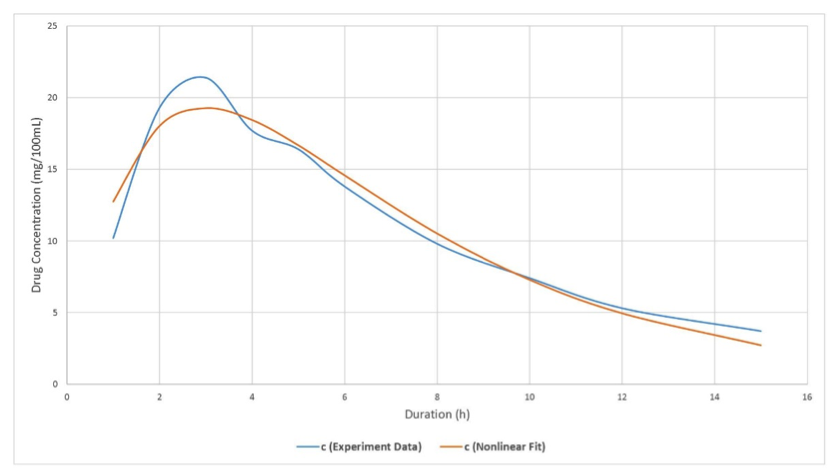Comparing experiment data and model fitted data, we find the model are in good agreement with experiments results.

### Discussion

It's necessary to monitor the variation of drug concentration in Body Tissue
Based on medical dynamics theory, we build a mathematical model to describe the drug time-dependent concentration change trend. Using this model, we can determine the drug concentration in blood from mathematical theory for various kinds of drug and different conditions. We use our experiments results to verify our model accuracy, and we find the model forecasting results are in good agreement with experiments results.
We believe our mathematic model, which gives a description of drug concentration change in body tissue, is able to be biological universal and reasonable for many cases. Due to the universal advantage of our model, this model can be regarded as one analysis reference for the drug metabolism research, moreover, medicinal development and clinical applications.

#### Style Switcher

12 Predefined Color Skins
Top Bar Color Layout Style Patterns for Boxed Version